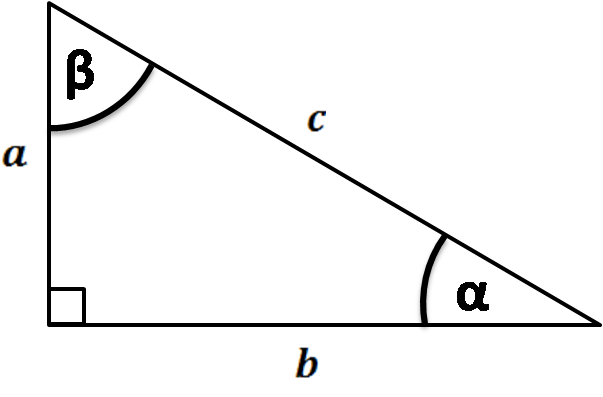# Tangent Angle Calculator

Created by Joanna Śmietańska, PhD candidate
Reviewed by Steven Wooding
Last updated: Jun 05, 2023

This quick tangent angle calculator will help you whenever you wonder how to find the tangent angle. It doesn't matter if the angle in your homework is given in degrees, radians, or pi radians. Read below to find out more helpful information about the tangent of an angle.

## How to find tangent angle?

In trigonometry or geometry, the tangent of an angle (simply denoted as tan) is the length of the side opposite the angle divided by the adjacent side. Note that this only applies to a right-angle triangle. Sounds complicated? Don't worry. Let's look at the image to help explain:If $\alpha$ is the angle between $b$ and $c$ sides of the triangle, then the tangent of an angle $\tan(\theta)$ is the ratio between opposite side $a$ and adjacent side $b$.

$\small{\tan(\theta) = \frac{a}{b}}$

Another definition states that tangent is the ratio of the sine function and cosine function, so tangent is the sin/cos ratio.

## Properties of tangent function

The tangent function is one of the main trigonometric functions. The graph of the tangent function has the peculiarity that it has no definite value at x = -π/2, π/2, -3π/2, 3π/2, 5π/2, etc. (or -90°, 90°, -270°, 270°, 450°, etc.).Unlike the sine or cosine, the tangent function has a period of $\pi$, so its values repeat after every π radian.

💡 Test it yourself! Try entering the angle π/2 (or 90° in degrees) into this tangent angle calculator and see what value it gives you.

## Example of how to calculate tangent of an angle

If you want to find the tangent of a given angle, enter the degree into our tangent angle calculator or follow these steps:

1. Identify the hypotenuse in your triangle. Usually, it is the longest side of the triangle.

2. Measure the two sides between which there is an angle and denote them as $a$ (opposite) and $b$ (adjacent), for example, $a = 21$ and $b = 8$.

3. Divide $a$ by $b$$a/b = 21/8$$= 2.63$. You will get a tangent of an angle.

## Other useful trigonometry calculators

Once you know how to use the tangent of angle calculator, check out our other trigonometric tools:

## FAQ

### Is tangent x/y?

No, the tangent of an angle is y/x. You may see the triangles, where r is the hypotenuse, x is the adjacent side at the angle, and y is the opposite side. But the tangent of an angle is the ratio of the opposite/adjacent sides, which in this case is y/x.

### What is the tangent of pi/4?

1. You can express the pi/4 in radians as 45° in degrees, and tan(45°) = 1. As the angle pi/4 lies between 0 and pi/2, the tangent function is positive and equal to 1.

Joanna Śmietańska, PhD candidate
Trigonometric function: Tangent
Angle
deg
People also viewed…

### Catenary curve

Discover the math behind a hanging rope with our catenary curve calculator.

### Foci of an ellipse

Use the foci of an ellipse calculator to find the x and y coordinates of an ellipse's foci, given its semi-major and semi-minor axes and center coordinates.

### Plant spacing

Planning out your garden? Try the plant spacing calculator.

### Secretary problem (Valentine's day)

Use the dating theory calculator to enhance your chances of picking the best lifetime partner.